Next: Evaluation procedure using the Up: Implementation Previous: Return code   Contents

Jacobian matrix

The user must provide a function which will compute the interval evaluation of the jacobian matrix of its particular functions for a given box. As for the function evaluation procedure we have chosen a syntax which shows the best compromise between program calls and interval calculation. The syntax of this function is:

INTERVAL_MATRIX IntervalGradient (int l1,int l2,INTERVAL_VECTOR & x)
• x: a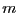dimensional interval vector which define the intervals for the unknowns
• l1,l2: the function must be able to return the interval evaluation of the component of the jacobian matrix at row l1 and column l2 i.e. the derivative of the function number l1 with respect to the variable number l2. The first row has number 1, the last n and the first column has number 1, the last m.
This procedure returns an interval matrix grad of size m X n in which grad(l1,l2) has been computed. This function should be written using the BIAS/Profil rules.

Note that if ALIAS_Store_Gradient has not been set to 0 we will store the simplified Jacobian matrix for each box. Indeed if for a given box B the interval evaluation of one element of the gradient has a constant sign (indicating a monotonic behavior of the function) setting the simplified jacobian matrix element to -1 or 1 allows to avoid unnecessary evaluation of the element of the Jacobian for the box resulting from a bisection of B as they will exhibit the same monotonic behavior. Although this idea may sound quite simple it has a very positive effect on the computation time. The name of the storage variable of the simplified jacobian is:

If a function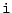is not differentiable you just set the value of grad(i,..) to the interval [-1e30,1e30]. It is important to define here a large interval as the program Compute_Interval_Function_Gradient that computes the interval evaluation of the function by using their derivatives uses also the Taylor evaluation based on the value of the derivatives: a small interval value of the derivatives may lead to a wrong function evaluation.
Note also that a convenient way to write the Gradient procedure is to use the procedure MakeJ offered by ALIAS-Maple (see the ALIAS-Maple manual). Take care also of the interval valuation problems of the element of the Jacobian (see section 2.1.1.3) which may be different from the one of the functions: for example if a function is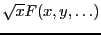it is interval-valuable as soon as the lower bound of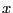is greater or equal to 0 although the gradient will involve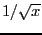which is not interval-valuable if the lower bound ofis equal to 0. ALIAS-Maple offers also the possibility to treat automatically the interval-valuation problems of the jacobian elements.